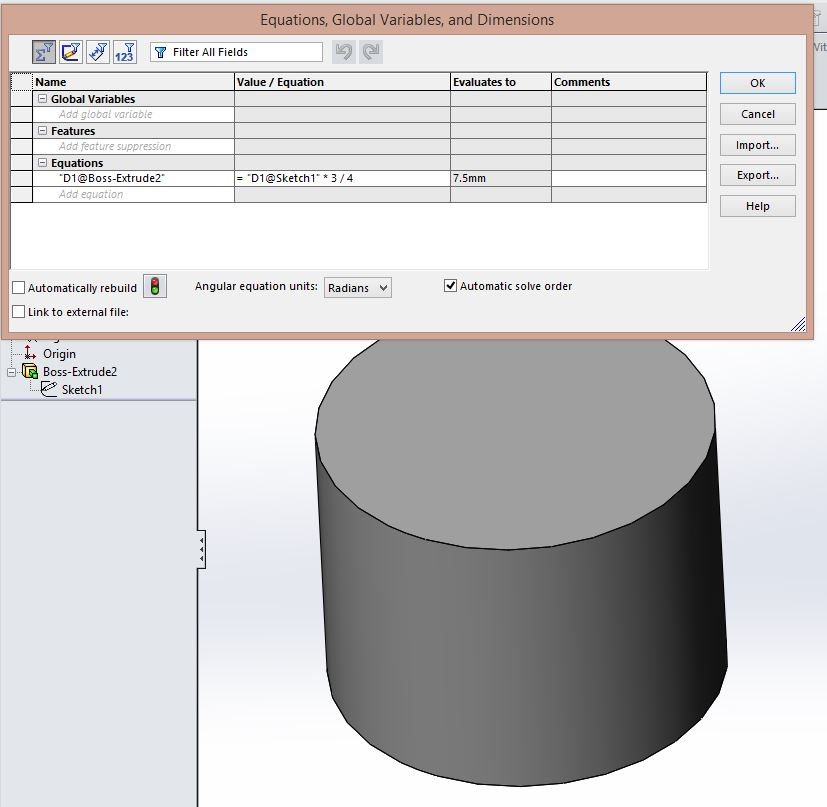# Screw conveyor modeling machine in Solidworks?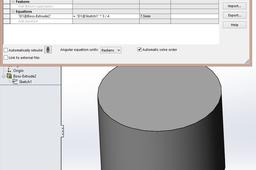Hello you are probably looking for 'equation' Feature of solidworks.
I can give you an easy example below:

Lets assume you want a cylinder which has an height of 3/4 th of its Diameter.
And you want to Change only the Diameter value and let the SW Change the rest.

1. ### Step 1:

Create your first sketch of the circle with a defined Dimension for the circle, 20 mm in this case.
SW saves this value as 'D1@Sketch1' which you haven't probably notice till now.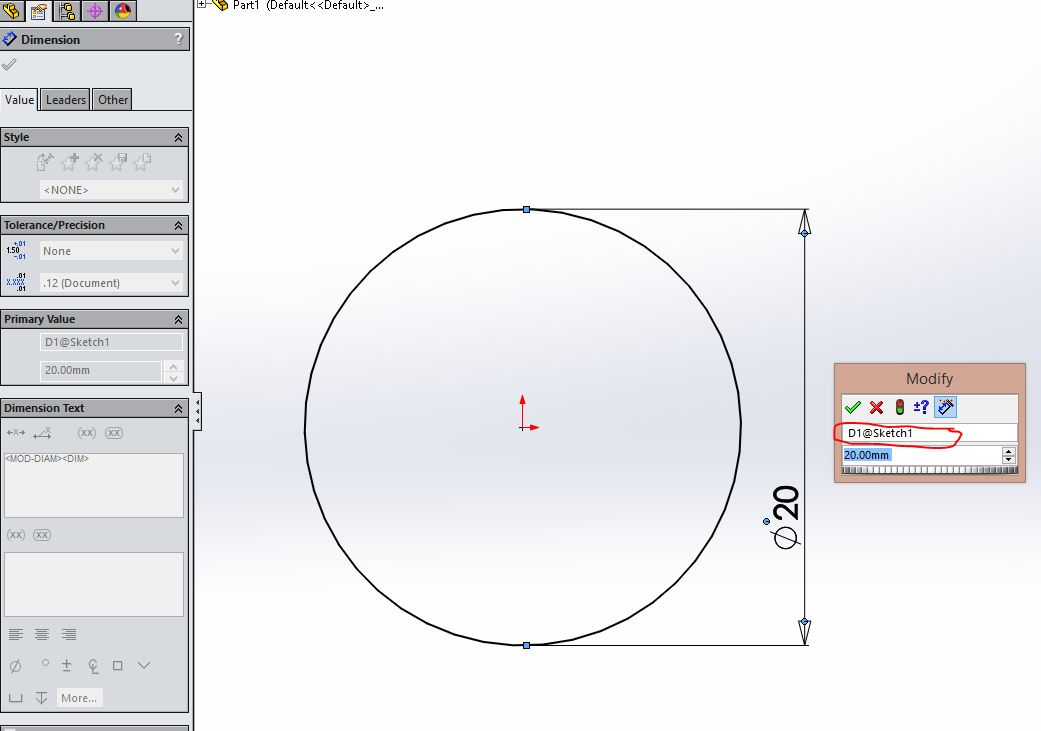2. ### Step 2:

Under the Boss-extrude Feature, instead of giving a specific value you can put an equation (just like Excel sheet).
For our case it is 'D1@Sketch1*3/4'.
This equation will automativcally Change the Boss-extrude value everytime you Change the Diameter in step 1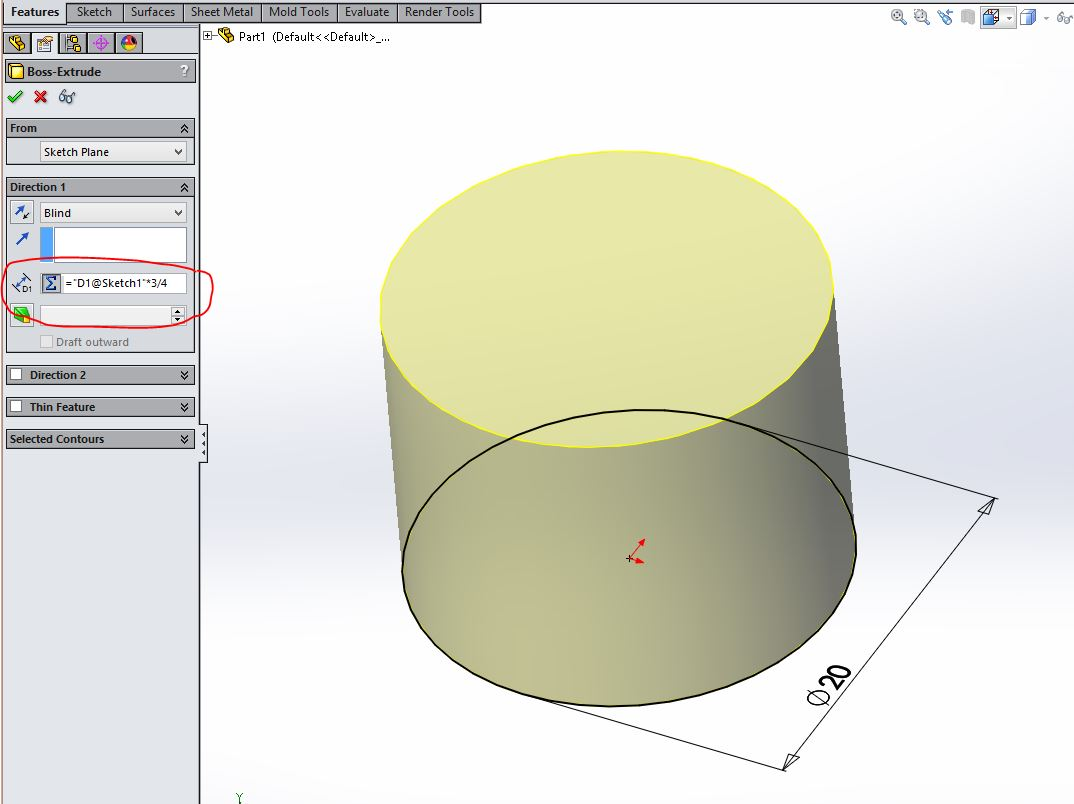3. ### Step 3:

If you want to Change the equation or any relationship between dimensions, just go under the 'Equation' tab in SW.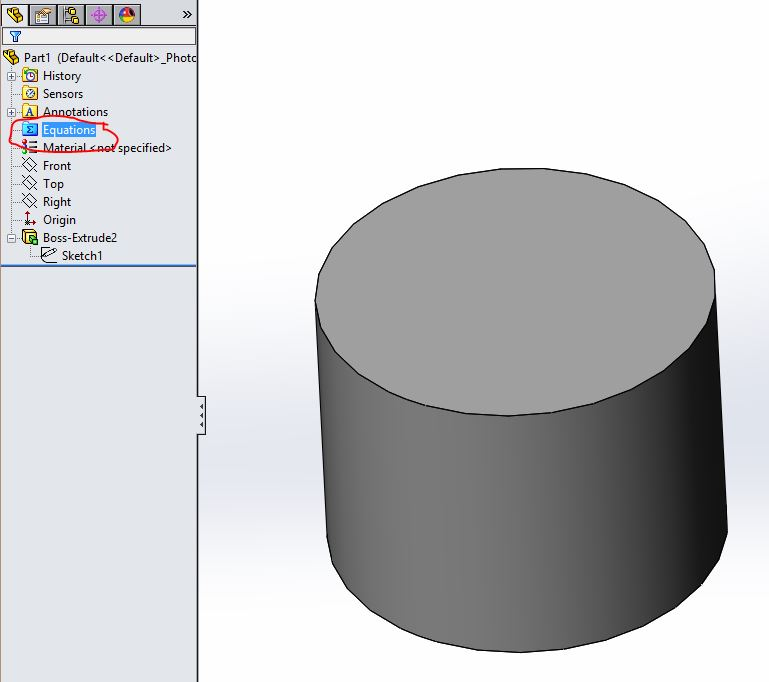4. ### Step 4:

You can Change, update or delete the relationship anytime you want from here.